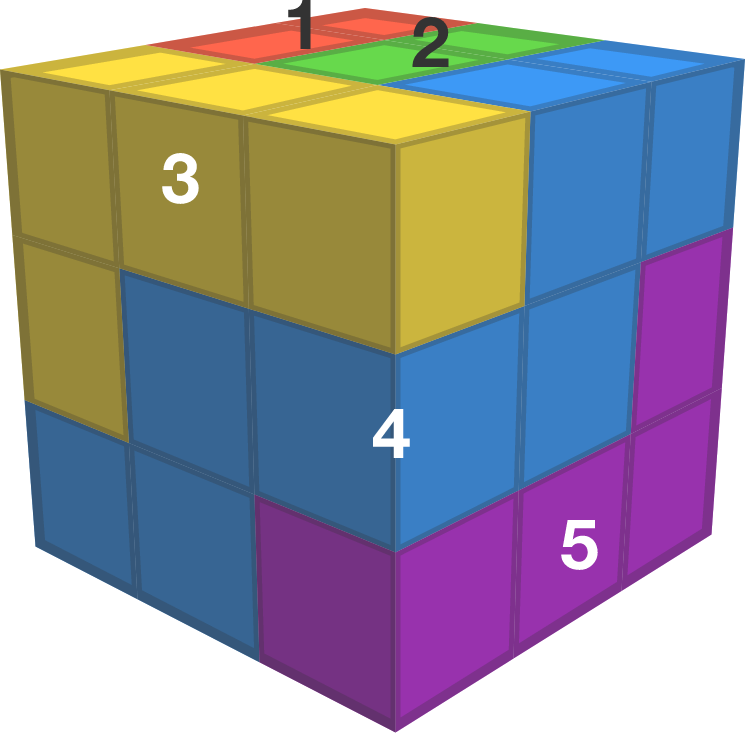# Cube Lattice Path Tilings

Probability Level 4

An NEU path (North, East, and Up path) in 3-D is a sequence of cubes, where each successive cube is either 1 unit north, east, or up. When represented as lattice points in $\mathbb{Z}^3$, the steps have size $(1,0,0), (0,1,0)$ or $(0,0,1)$.

The goal is to tile a $27 \times 27 \times 27$ cube using NEU paths which fit entirely within the cube and do not overlap each other. What is the fewest number of NEU paths that are needed?An NEU path tiling of a $3 \times 3 \times 3$ cube with 7 paths (paths 6 and 7 are hidden from view)

As an explicit example, the minimal tiling of a $3 \times 3 \times 3$ cube with NEU paths requires 7 paths. An example is shown to the right, but 2 paths are hidden from view.

×

Problem Loading...

Note Loading...

Set Loading...